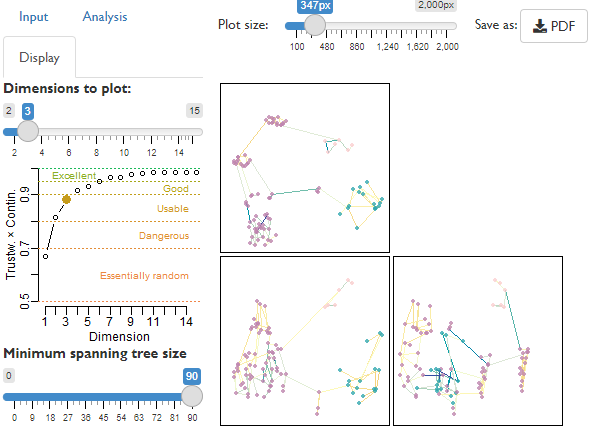‘TreeDist’ is an R package that implements a suite of metrics that quantify the topological distance between pairs of unweighted phylogenetic trees. It also includes a simple ‘Shiny’ application to allow the visualization of distance-based tree spaces, and functions to calculate the information content of trees and splits.

‘TreeDist’ primarily employs metrics in the category of ‘generalized Robinson–Foulds distances’: they are based on comparing splits (bipartitions) between trees, and thus reflect the relationship data within trees, with no reference to branch lengths.

## Generalized RF distances

The Robinson-Foulds distance simply tallies the number of non-trivial splits (sometimes inaccurately termed clades, nodes or edges) that occur in both trees – any splits that are not perfectly identical contribute one point to the distance score of zero, however similar or different they are. By overlooking potential similarities between almost-identical splits, this conservative approach has undesirable properties.

‘Generalized’ RF metrics generate matchings that pair splits in one tree with similar splits in the other. Each pair of splits is assigned a similarity score; the sum of these scores in the optimal matching then quantifies the similarity between two trees.

Different ways of calculating the the similarity between a pair of splits lead to different tree distance metrics, implemented in the functions below:

The package also implements the variation of the path distance proposed by Kendal and Colijn (2016) (function KendallColijn()), approximations of the Nearest-Neighbour Interchange (NNI) distance (function NNIDist(); following Li et al. (1996)), and calculates the size (function MASTSize()) and information content (function MASTInfo()) of the Maximum Agreement Subtree.

For an implementation of the Tree Bisection and Reconnection (TBR) distance, see the package ‘TBRDist’.

# Installation

Install and load the library from CRAN as follows:

install.packages('TreeDist')
library('TreeDist')

You can install the development version of the package with:

if(!require("curl")) install.packages("curl")
if(!require("remotes")) install.packages("remotes")
remotes::install_github("ms609/TreeDist")

# Tree space analysis

Construct tree spaces and readily visualize projected landscapes, avoiding common analytical pitfalls (Smith, 2022), using the inbuilt graphical user interface (Shiny GUI):

TreeDist::MapTrees()image

Serious analysts should consult the vignette for a command-line interface.

# Documentation

Other R packages implementing tree distance functions include:

• ape’:
• cophenetic.phylo(): Cophenetic distance
• dist.topo(): Path (topological) distance, Robinson-Foulds distance.
• phangorn
• treedist(): Path, Robinson-Foulds and approximate SPR distances.
• Quartet’: Triplet and Quartet distances, using the tqDist algorithm.
• TBRDist’: TBR and SPR distances on unrooted trees, using the ‘uspr’ C library.
• treespace’: Kendall-Colijn distance and tree space visualizations.
• distory’ (unmaintained): Geodesic distance

Please note that the ‘TreeDist’ project is released with a Contributor Code of Conduct. By contributing to this project, you agree to abide by its terms.